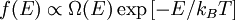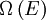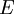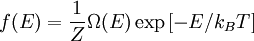Boltzmann distribution$f(E) \propto \Omega(E) \exp \left[ - E/k_B T \right]$,
where$\Omega \left( E \right)$ is the degeneracy of the energy$E$; leading to$f(E) = \frac{1}{Z} \Omega(E) \exp \left[ -E/k_B T \right]$.
where$k_B$ is the Boltzmann constant, T is the temperature, and the normalization constant Z is the partition function of the system.